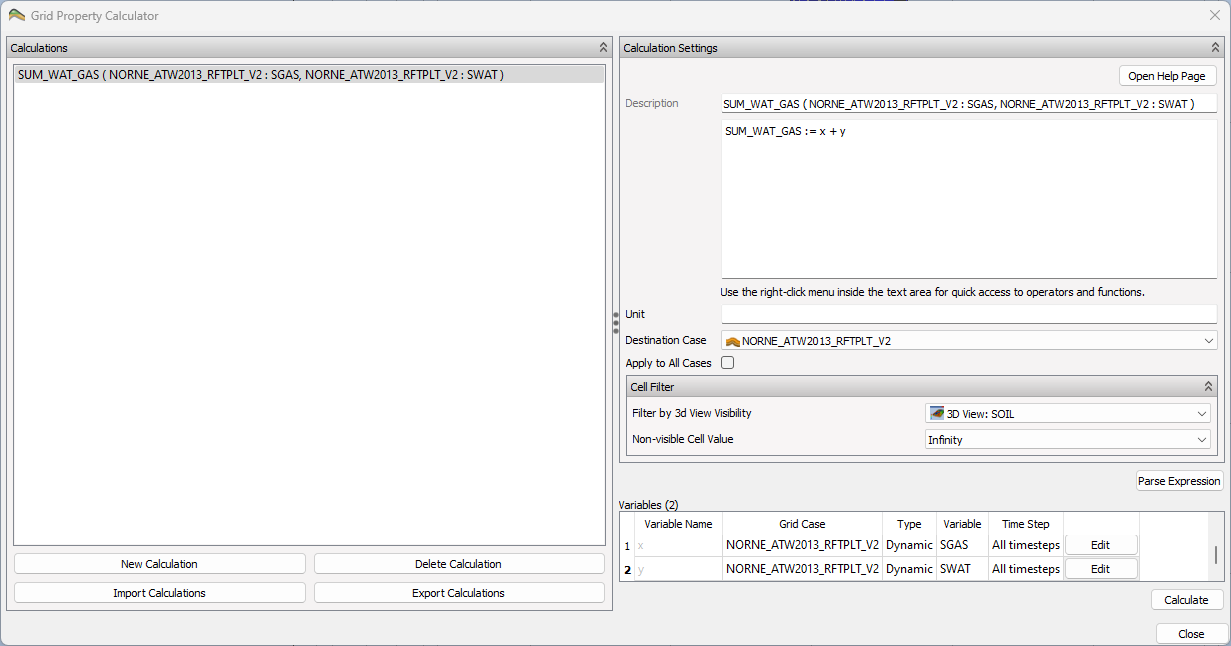# Grid Property Calculator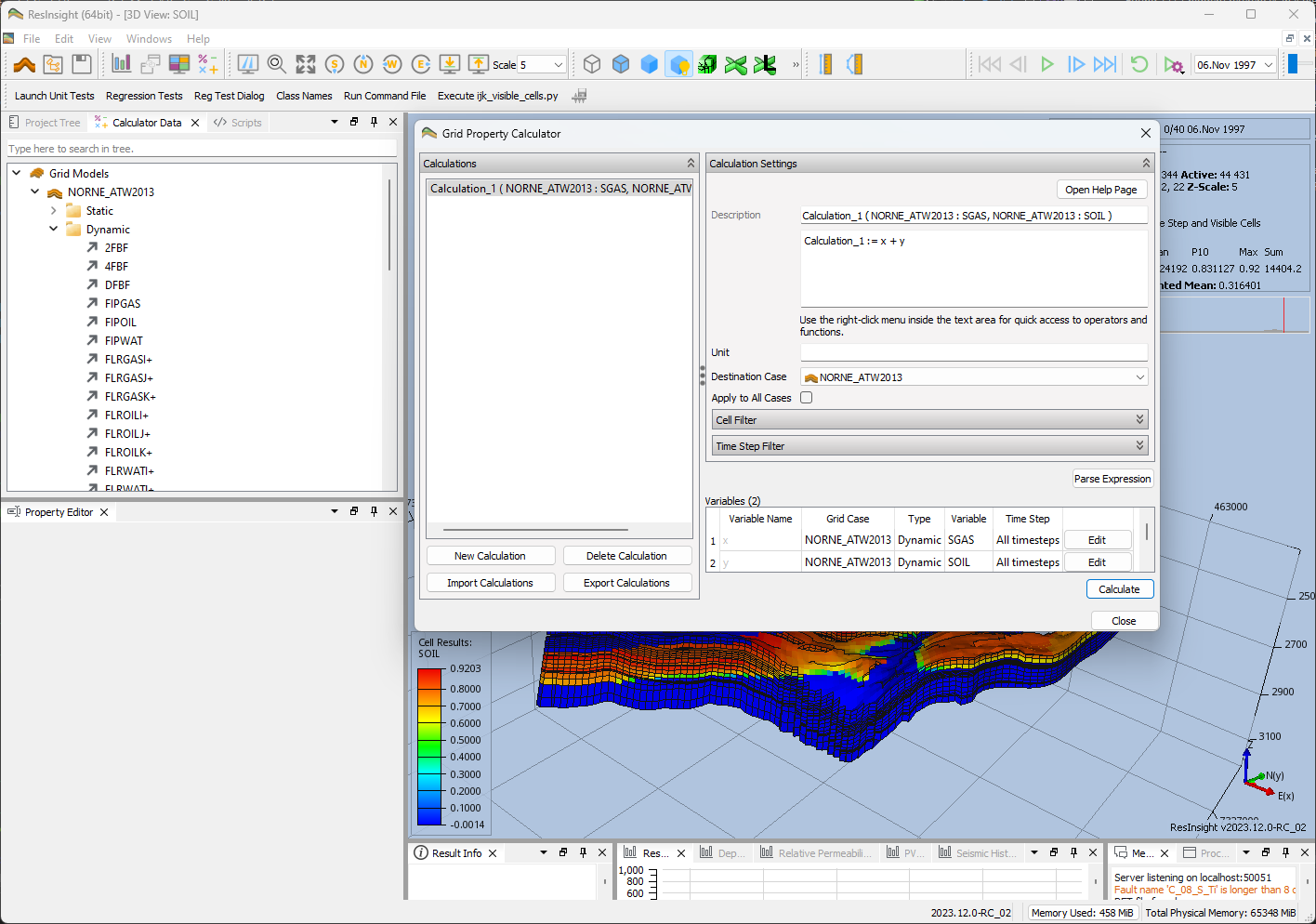ResInsight offers a built-in property calculator for grid parameters. The Grid Property Calculator enables arithmetic expressions to be parsed and calculated for visualization purposes.

More details on mathematical operations are described in Calculator Expressions

## Invoking the Grid Property Calculator

Invoke the the Grid Property Calculator by pressing the calculator iconin the menu bar or by right-clicking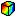Cell Result in Project Tree.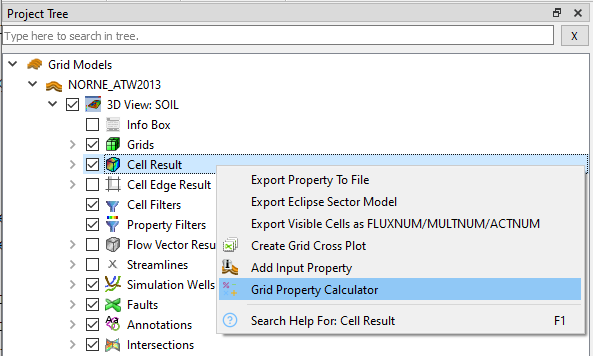## Example using Grid Property Calculator

As shown, the Grid Property Calculator is invoked by right-clickingCell Result in Project Tree. As seen below, the Grid Property Calculator is per default prefilled with the simple summation Calculation_1 := x + y. This simple summation suffices for exemplifying its use. However, you may change name of the calculation and add additional variables and arithmetic operators in the Expression field.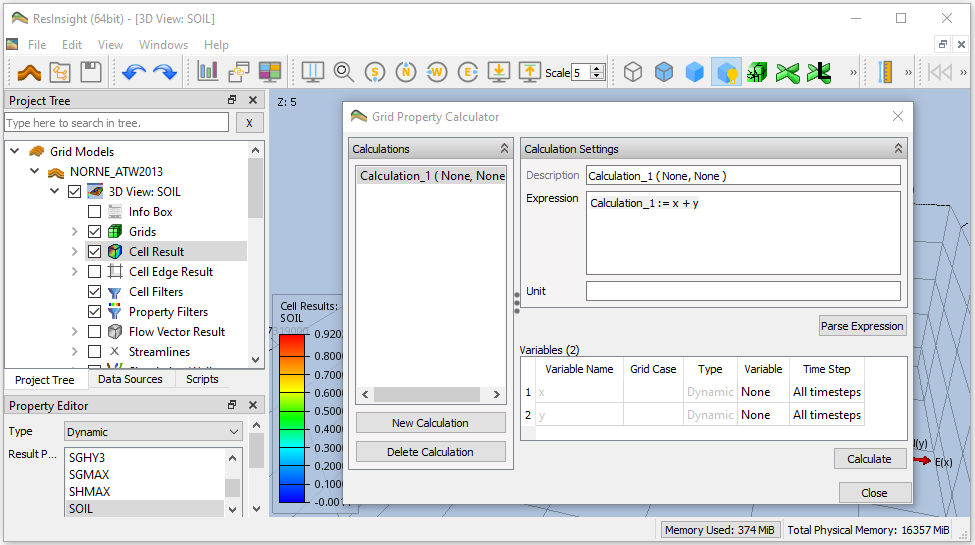The next step is to define the variables x and y. An easy way to define each variable is to drag and drop properties from Data Sources. See screenshot below for drag and drop of SOIL from Data Sources to the row of variable x in Grid Property Calculator (emphasized in red). Continue with drag and drop of SGAS to define y.Finally, press Calculate to perform the actual calculation.

The calculated result is accessable via Property Editor as Type Generated Result Property.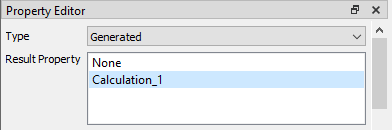## Reference Procedure using Grid Property Calculator

1. Invoke the the Grid Property Calculator by right-clickingCell Result in Project Tree.
2. Define the expression for calculation by the involved variables and arithmetic operators
• Type the name of calculation, required variables and aritmetic operators into Expression
• Press button Parse Expression to verify the expression and referrals to actual variables
3. Define each actual variable referred by the expression, either manually or by drag and drop from Data Sources
4. Press Calculate to perform the actual calculation
5. Access the calculated result in Property Editor as Type Generated Result Property